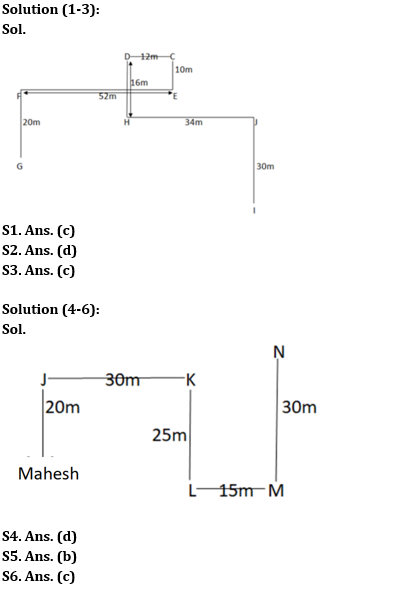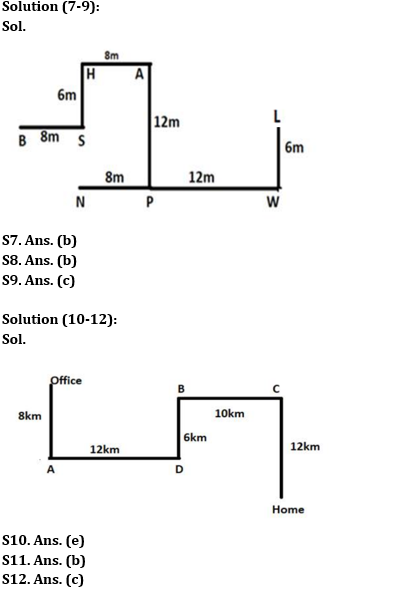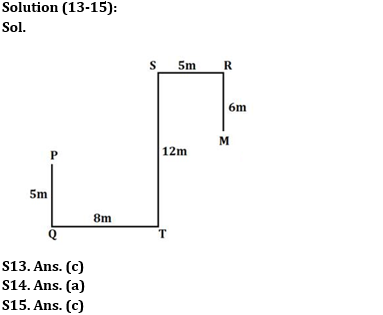Latest Banking jobs   »   Reasoning Ability Quiz For SBI Clerk...

# Reasoning Ability Quiz For SBI Clerk Prelims 2022- 28th April

Directions (1-3): Study the following data carefully and answer the questions accordingly.
Point C is 12m east of point D, which is 16m north of point H. Point F is 52m west of point E. Point I is 30m south of point J. Point E is 10m south of point C. Point J is 34m east of point H. Point F is 20m north of point G.

Q1. Point C is in which direction with respect to point F?
(a) South
(b) South-West
(c) North-East
(d) West
(e) None of these

Q2. Which of the following points is not in the north-east of point G?
(a) J
(b) C
(c) D
(d) I
(e) None of these

Q3. Point J is in which direction with respect to point D?
(a) South-West
(b) North-West
(c) South-East
(d) North
(e) Can’t be determined

Directions (4-6): Study the data carefully and answer the questions accordingly.
Mahesh starts walking towards north and covers a distance of 20m to reach point J. Now, he took a right turn to reach point K after a distance of 30m. Then, he turned right and covers a distance of 25m to reach point L. From here, he turned towards east and covers a distance of 15m to reach point M. Finally, he turned left and reached point N after a distance of 30m.

Q4. Point N is in which direction of point J?
(a) East
(b) North
(c) South-West
(d) North-East
(e) None of these

Q5. What is the shortest distance between Mahesh’s starting point and point M?
(a) 23m
(b) 5√82m
(c) 31m
(d) 6√72m
(e) None of these

Q6. Point M is in which direction with respect to point J?
(a) East
(b) North
(c) South-East
(d) North-East
(e) None of these

Directions (7-9): Read the following information carefully and answer the questions which follow.
Point L is 6m to the north of point W which is 12m to the east of point P. Point N is 8m to the west of Point P. Point P is 12m to the south of Point A. Point A is 8m to the east of Point H. Point S is 6m to the south of Point H. Point B is 8m to the west of Point S.

Q7. What is the direction of Point W with respect to Point B?
(a) North
(b) South-east
(c) South-west
(d) North-west
(e) None of these

Q8. What is the shortest distance between Point B and Point H and in which direction is Point A with respect to Point H?
(a) 10m, West
(b) 10m, East
(c) 12m, West
(d) 13m, east
(e) None of these

Q9. What is the direction of point N and point W with respect to point P respectively?
(a) North-west and North-east
(b) North-west and East
(c) West and East
(d) East and West
(e) None of these

Directions (10-12): Read the following information carefully and answer the questions which follow.
Aniket started walking from his office towards south and walked for 8km and reached Point A, then he turned towards his left and walked for 12km and reached Point D, then he walked 6km towards north and reached Point B after that he turned towards his right and walked for 10km and reached Point C, finally he turned towards south and walked for 12km and reached his home.

Q10. If point A is 9km to the north of point F, then what is the shortest distance between point F and point D?
(a) 12km
(b) 14km
(c) 17km
(d) 13km
(e) 15km

Q11. What is the direction of Point A with respect to final position of Aniket?
(a) North-east
(b) North-west
(c) South-west
(d) East
(e) None of these

Q12. If point E is 4km to the west of point D, then what is the total distance between point C and point E?
(a) 22km
(b) 24km
(c) 20km
(d) 18km
(e) None of these

Directions (13-15): Study the following data carefully and answer the questions accordingly.
Point P is 5m to the north of Point Q. Point S is 5m west of Point R. Point M is 6m south of Point R. Point T is 12m south of Point S. Point Q is 8m west of Point T.

Q13. What is the shortest distance between Point R and Point T?
(a) 12m
(b) 14m
(c) 13m
(d) 18m
(e) None of these

Q14. What is the direction of Point M with respect to Point P?
(a) North-east
(b) North-west
(c) South-west
(d) East
(e) None of these

Q15. What is the total distance between P and S?
(a) 23m
(b) 24m
(c) 25m
(d) 28m
(e) None of these

Solutions#### Congratulations!Download Hindu Review of October 2021: Free PDF